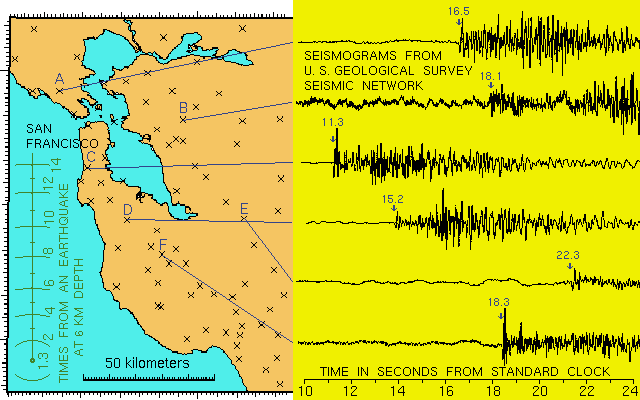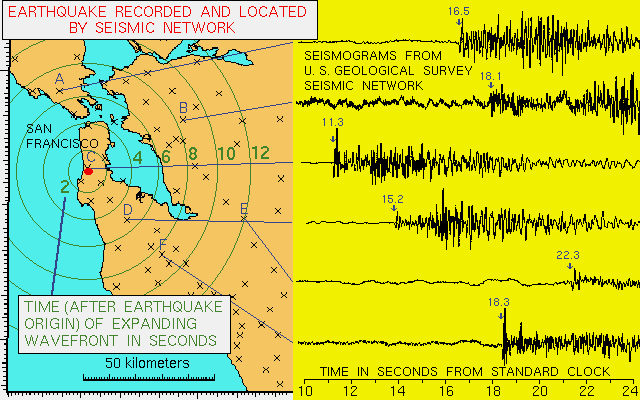An official website of the United States government

# How do seismologists locate an earthquake?

When an earthquake occurs, one of the first questions is "where was it?" The location may tell us what fault it was on and where damage (if any) most likely occurred.

Unfortunately, Earth is not transparent and we can't just see or photograph the earthquake disturbance like meteorologists can photograph clouds. When an earthquake occurs, it generates an expanding wavefront from the earthquake hypocenter at a speed of several kilometers per second.

We observe earthquakes with a network of seismometers on the earth's surface. The ground motion at each seismometer is amplified and recorded electronically at a central recording site. As the wavefront expands from the earthquake, it reaches more distant seismic stations. When an earthquake occurs, we observe the times at which the wavefront passes each station. We must find the unknown earthquake source knowing these wave arrival times. Here is a map of U.S. Geological Survey seismic stations in the San Francisco Bay Area and 6 seismograms from an earthquake:Map of U.S. Geological Survey seismic stations in the San Francisco Bay Area and 6 seismograms from an earthquake (public domain).

We want to find the location, depth and origin time of an earthquake whose waves arrive at the times measured on each seismograms. We want a straightforward and general procedure that we can also program in a computer. The procedure is simple to state: guess a location, depth and origin time; compare the predicted arrival times of the wave from your guessed location with the observed times at each station; then move the location a little in the direction that reduces the difference between the observed and calculated times. Then repeat this procedure, each time getting closer to the actual earthquake location and fitting the observed times a little better. Quit when your adjustments have become small enough and when the fit to the observed wave arrival times is close enough.

You can try to fit an earthquake location on the map just to see how the procedure goes. Note that the earthquake arrives first on station C, so C is a good first guess for the location. Many earthquakes in California occur between 2 and 12 kilometers depth and we will guess a 6 kilometer depth. The origin time should be a few seconds before the time of the wave at the first station. Let's guess an origin time of 10 seconds, measured on the same clock that made the time scale at the bottom of the figure and timed the seismograms. Then we can list the tentative travel times by subtracting the origin time from the observed arrival times:

station....................... A        B         C         D         E         F

observed time..........  16.5    17.8    11.3    15.2    22.3    18.3

tentative travel time... 6.5     7.8      1.3      5.2      12.3    8.3

Note the scale at the left of the figure. It shows travel times for waves from an earthquake at a depth of 6 kilometers. The scale starts at 1.3 seconds because the wave reaches the surface 1.3 seconds after the earthquake origin time. You can make a tracing of the scale and move the earthquake on the map until the tentative travel times match the travel times from the scale. Where do you think the earthquake was? Are the times for each station systematically early or late, requiring a shift in the origin time?Map of U.S. Geological Survey seismic stations in the San Francisco Bay Area and derived location of earthquake (public domain).

The earthquake was near station C. The depth was about 6 km and the origin time was about 10 seconds. (We guessed very well!) A real magnitude 3.4 earthquake occurred at this location on April 29, 1992. It was felt by many people who were sitting or at rest.

Mathematically, the problem is solved by setting up a system of linear equations, one for each station. The equations express the difference between the observed arrival times and those calculated from the previous (or initial) hypocenter, in terms of small steps in the 3 hypocentral coordinates and the origin time. We must also have a mathematical model of the crustal velocities (in kilometers per second) under the seismic network to calculate the travel times of waves from an earthquake at a given depth to a station at a given distance. The system of linear equations is solved by the method of least squares which minimizes the sum of the squares of the differences between the observed and calculated arrival times. The process begins with an initial guessed hypocenter, performs several hypocentral adjustments each found by a least squares solution to the equations, and iterates to a hypocenter that best fits the observed set of wave arrival times at the stations of the seismic network.